All submissions of the EM system will be redirected to Online Manuscript Submission System. Authors are requested to submit articles directly to Online Manuscript Submission System of respective journal.

Original Article
, Volume: 12( 2)

# Theoretical Studies on Effect of Substituent on Aromaticity of Highly Substituted Benzene Rings

*Correspondence:
Shamlouei HR
Chemistry Department, Lorestan
University, Khorram Abad, Lorestan, I.R. Iran
Tel: 986616200610
Fax: 986616200612
E-mail: [email protected]

Received Date: May 25, 2017 Accepted Date: July 28, 2017 Published Date: August 04, 2017

Citation: Shamlouei HR, Tahmaszadeh F, Alavi M. Theoretical Studies on Effect of Substituent on Aromaticity of Highly Substituted Benzene Rings. Phys Chem Ind J. 2017;12(2):111

### Abstract

In this research, effect of electronegative F, Cl, NO2 and CF3 substituents on aromaticity of highly substituted benzene molecules is studied. NICS, ASE, HOMA and HOMO-LUMO energy gaps were the indices which are applied to show effect of substituents. In addition, sigma and pi-electron donor acceptors are calculated for all the substituted molecules. Additionally, total electron donor acceptor tEDA was proposed as a new descriptor which was the sum of two previously mentioned descriptors and show total electrons. The trend of results is different for all methods. For instance the behavior of NICS is contrariwise to ASE and HOMO-LUMO energy gaps results and HOMA index calculation provided no valuable information. As other results, it was shown that the calculated NICS in center of rings was changed not only by ring current but also altered with the presence of other atoms fields. The isomerization effect of the aromaticity also was studied and it was shown that the energy, ASE, and HOMA indices adapted to tEDA descriptors. Deficiency of NICS and HOMA methods in predicting the substituent effects illuminated in this research which the NICS deficiency is explained by the fact that the chemical shift of nucleus was resulted from two sources: ring current and other atoms presence.

### Keywords

Aromaticity; Substituent effects; NICS; ASE; HOMA; HOMO-LUMO gap

### Introduction

Aromaticity is the characteristic which exists in conjugated cycles and is produced when cyclic delocalization and resonance occur [1-3]. This property resulting from cyclic conjugation is an important concept in hemistry [4-5]. According to the properties, aromatic compounds have some characteristics such as:

1. Electrophilic aromatic substitution as one of their chemical reactions,

2. Occurrence of bond length equalization due to cyclic delocalization in aromatic molecules,

3. Having large resonance energy and enhanced stability,

4. Existence of ring current in these compounds which induces a magnetic field around them .

Aromaticity is one of the features which controls stability and reactivity of unsaturated cyclic and polycyclic compounds and has a key role in many reactions that occur in biological systems . Aromaticity of rings is evaluated on the basis of some energetic, geometric, electronic and magnetic criteria. Nucleus independent chemical shift (NICS) is another criterion used to show aromaticity of rings [7,8]. Aromatic stability energy (ASE) is one of the energetic criteria which is used to interpret aromaticity . Hydrogenation enthalpies of aromatic molecules may be related to ASE .

A relatively large energy gap between higher occupied molecular orbital (HOMO) and lower unoccupied molecular orbital (LUMO) is one of the characteristics of aromatic compounds , which corresponds to lower reactivity of aromatic compounds to electrophilic reagents. Chemical hardness (?) can be determined from the energy of these two orbitals according to eqn. (1) :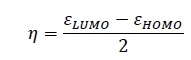(1)

In whichand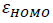correspond to energy of LUMO and HOMO, respectively. So, hardness can be account as aromaticity index. Harmonic oscillator model for aromaticity (HOMA) is another index which corresponds to structural criterion of aromaticity .

Krygowski, et al. showed that the substituents with electron donating character will stabilize the system and effect of substituent was considered as new descriptors σEDA and πEDA which correspond to sigma and pi electron donor acceptors , respectively. A new descriptor can be defined as total electron donor acceptor or tEDA which interprets total electron attraction form or release to rings.

The aim of this research was to study effect of electronegative substitutions on aromaticity. Additionally, performances of various indices in predicting aromaticity were compared. For this reason, all the indices mentioned for benzene substituted with different numbers of F, Cl, NO2 and CF3 substitutions were calculated.

To study substituent effect on aromaticity, benzene molecule was selected. Then, H of benzene was replaced with different numbers of electronegative substituents such as F, Cl, NO2 and CF3. Structure of molecules was optimized by B3LYP method, 6-311++G (d, p) basis sets, and Gaussian 03w program. After optimizing structure of rings, natural population analysis (NPA) was done to calculate σEDA and πEDA and consequently tEDA (total electron donor acceptor) descriptors. Then, Guess Ghost atoms were located in the center of the optimized structure of rings from zero to about 3.0 Angstrom and the NMR or magnetic field above the rings (NICS) were calculated by HF method and the basis set which was previously mentioned. In Figure. 1 , schematics of benzene molecule along with Guess Ghost atoms which were used in NICS calculation for benzene molecule are demonstrated.

Figure 1: Schematic of Guess Ghost atoms located above benzene molecule to calculate the magnetic field.

Enthalpy of hydrogenation of aromatic ring which is the key for estimating the aromaticity stabilization energy (ASE) was calculated. The hydrogenation reaction of benzene molecule is illustrated in eqn. (2).(2)

For calculation the enthalpy of reaction described in eqn. (2), the structure and energy parameters of reactants and products for all substituted aromatic molecules were calculated by B3LYP method, 6-311++G (d, p) basis sets.

### Results and Discussion

Structural optimization and natural population analysis

First, structures of all benzene molecules were substituted with different substituents, H2 molecule and product of hydrogenation reaction of substituted benzene molecule by previously mentioned basis set and method. It was shown that the different isomers of 2-fluoro, 3-fluoro and 4-fluoro benzene had different energy. The difference in their energy may be subsequent of effect of symmetry in aromaticity. Then their natural bonding orbitals for each molecule were calculated and natural atomic orbital occupancies were used to calculate σEDA and πEDA of these substituted molecules according to the method presented by Dobrowolski . By summing σEDA and πEDA, tEDA was calculated. As represented by Dobrowolski, some of the substituents such as F were σ-donor, but π-acceptor. It was shown that the calculated σEDA and πEDA and consequently tEDA are additive and, with increasing the number of substituents, their amount increased. In Table 1, the calculated energy, σEDA and πEDA and tEDA are presented for all the substitutes.

Number of substituents F Cl NO2 CF3
σEDA πEDA tEDA σEDA πEDA tEDA σEDA πEDA tEDA σEDA πEDA tEDA
1 -0.583 0.068 -0.515 -0.248 0.068 -0.180 -0.301 -0.066 -0.368 -0.121 -0.019 -0.141
2 -1.167 0.133 -1.035 -0.478 0.136 -0.342 -0.608 -0.100 -0.708 -0.230 -0.034 -0.264
3 -1.762 0.208 -1.554 -0.703 0.220 -0.484 -0.911 -0.131 -1.041 -0.338 -0.048 -0.386
4 -2.356 0.279 -2.077 -0.927 0.304 -0.623 -1.356 -0.024 -1.380 -0.460 -0.042 -0.502
5 -3.921 0.355 -3.566 -1.148 0.404 -0.745 -1.717 0.029 -1.688 -0.614 -0.012 -0.626
6 -3.538 0.448 -3.090 -1.368 0.502 -0.866 -2.087 0.094 -1.993 -0.936 -0.900 -1.835

Table 1. Calculated σEDA, πEDA and tEDA for different numbers of substituents.

In Table 1 it was shown that the substituent had extensive effect on electron population in the benzene ring. For example existence of F substituent instead of hydrogen atom, withdraw the electron by sigma bonding however it releases electron to ring by pi bonding. Totally the F substituent had withdrawing effect on electron population which may be predictable from higher electronegativity of F in comparison to H. In Table 1, it was shown that the substituent effects were additive properties and its absolute values increases as the number of substituent increases.

It is clear that substituent effect can be concisely studied by considering σEDA and πEDA and tEDA which is obviously presented in Table 1.

NICS evaluation for substituted benzene molecules

Afterward, the magnetic fields above the substituted benzene molecules were calculated. In Figure. 2, plot of the negative of calculated NICS as a function of distance from center of rings are presented for F.

Figure 2: Plots of calculated NICS as a function of distance from center C6H6 substituted with different numbers of F.

In Figure. 3 it was shown that the negative of calculated NICS in various distances from center of ring for fluorinated benzene completely differ from unfluorinated molecule. Figure. 2 proposed that the NICS values, which correspond to magnetic field above the ring, highly depend on presence of fluorine atoms. Figures. 3-5, plots of the negative calculated NICS as a function of distance from center of rings are presented for Cl, NO2 and CF3 substituents, respectively.

Figure 3: Plots of calculated NICS as a function of distance from center C6H6 substituted with different numbers of Cl.

Figure 4: Plots of calculated NICS as a function of distance from center C6H6 substituted with different numbers of NO2.

Figure 5: Plots of calculated NICS as a function of distance from center C6H6 substituted with different numbers of CF3.

Similar to Figure. 2, Figures. 3-5 show that plots of the negative of calculated NICS for the substituted benzene were different and, it was common that with increasing the number of substituents, the negative of NICS values increased. For clarifying the behavior of NICS versus the number of substituent, the negative of NICS values in the center of ring (NICS-0) were calculated and the results confirmed that, with increasing the number of substituent, the negative of NICS (0) was increased.

As stated above, the substituent effects could be revealed by tEDA. In Figures. 6-9, the negative of NICS-0 for substituted benzene as a function of the calculated tEDA for the substituents is illustrated for F, Cl, NO2 and CF3, respectively.

Figure 6: NICS-0 for substituted benzene as a function of the calculated tEDA for the F substituents.

Figure 7: NICS-0 for substituted benzene as a function of the calculated tEDA for the Cl substituents.

Figure 8: NICS-0 for substituted benzene as a function of the calculated tEDA for the NO2 substituents.

Figure 9: NICS-0 for substituted benzene as a function of the calculated tEDA for the CF3 substituents.

In Figures. 6-9, it is demonstrated that, with increasing the number of substituents and consequently decreasing tEDA descriptors, the negative of NICS value increased. It might be considerable that the used substituents, especially F, had higher electronegativity in comparison to H atoms. This fact was illustrated by considering σEDA, πEDA and tEDA. Total electron transferred to the ring can be briefly counted by tEDA. All the substituted rings had negative values of tEDA descriptor; so, the substituents would decrease the available electron density in aromatic ring. It was expected that, as electron density in the ring decreased, its above magnetic field would decrease. Additionally, as a consequence of replacing H atoms of benzene, the symmetry of ring might decrease and therefore aromaticity had to be decreased. This expectation was not confirmed by the computational results. The result which is illustrated in Figures. 6-9showed that, as the number of substituents increased or, when the tEDA descriptor decreased, the negative of calculated NICS increased. This result was in contrast to the anticipation.

Difference between negative of NICS calculation and prediction may be account by considering this fact that the substituents had an amplifying effect on the calculated magnetic field. In other words, in the presence of other atoms attaching to the ring, the calculated magnetic field was exaggerated by the field induced by these atoms. To inquire this fact, the magnetic field inside the cyclohexane and substituted cyclohexane were calculated to show effect of field of substituents on negative of calculated NICS in the absence of aromaticity. In Table 2 calculated NICS in center of cyclohexane rings were shown.

Formula NICS (0) Formula NICS (0)
C6H12 -1.4596 C6H8F4 -6.7669
C6H11F -3.8951 C6H7F5 -6.9879
C6H10F2 -4.8832 C6H6F6 -7.3961
C6H9F3 -6.047

Table 2. Values of NICS (0) in the center of cyclohexane molecule substituted with different number of -F group.

It is evident from Table 2, that the calculated magnetic field in the center of the ring is affected by the field induced by other atoms. Consequently, it was concluded that the calculated NICS at least was originated from two sources: one from the ring current and one from other atoms presence.

Enthalpy of hydrogenation or aromatic stabilization energy (ASE) calculation

The aromatic stabilization energy (ASE) is the amount of stabilization energy of aromatic systems due to aromaticity. If the ASE will be large, the enthalpy of hydrogenation must decrease. So, the value of enthalpy of hydrogenation may be the representative of ASE. In order to test validity of the results obtained in Figures. 6-9, enthalpy of hydrogenation was calculated for all the substituted benzene molecules. Interestingly, it was illustrated that, as the number of substituents increased, their enthalpy of hydrogenation increased. As mentioned previously, the substituted effect was abstracted in tEDA; so, results of enthalpy of hydrogenation of the substituent benzene molecules could be plotted as its function. In Figures. 10-13, enthalpy of hydrogenation of the substituent benzene molecules as a function of calculated tEDA is demonstrated for F, Cl, NO2 and CF3, respectively.

Figure 10: Enthalpy of hydrogenation of the substituent benzene molecules as a function of calculated tEDA for F substituents.

Figure 11: Enthalpy of hydrogenation of the substituent benzene molecules as a function of calculated tEDA for Cl substituents.

Figure 12: Enthalpy of hydrogenation of the substituent benzene molecules as a function of calculated tEDA for NO2 substituents.

Figure 13: Enthalpy of hydrogenation of the substituent benzene molecules as a function of calculated tEDA for CF3 substituents.

In Figures. 10-13, it was shown that, with increasing the number of substituents and consequently decreasing electron density in the ring, enthalpy of hydrogenation increased; i.e. with increasing the number of substituents, ASE (aromaticity) of rings decreased. These results showed a different trend from NICS calculation.

What is the origin of inconsistency in the results? According to the prediction, electronegative substituents might draw electron density from rings and therefore reduce its aromaticity. So, it seems that results of ASE calculation were more reliable than NICS calculation because of the fact that the NICS value affected not only by ring current but also exaggerated by presence of other atoms.

HOMO-LUMO energy gap

As stated in the introduction, aromatic compounds might have a large HOMO-LUMO gap and consequently high hardness. According to eqn. (1), hardness of each substituted molecule was calculated and plotted as a function of tEDA values which were previously determined. In Figures. 14-17, plots of the calculated hardness are illustrated.

Figure 14: The calculated chemical hardness as a function of tEDA for benzene molecule substituted with different numbers of F.

Figure 15: The calculated chemical hardness as a function of tEDA for benzene molecule substituted with different numbers of Cl.

Figure 16: The calculated chemical hardness as a function of tEDA for benzene molecule substituted with different numbers of NO2.

Figure 17: The calculated chemical hardness as a function of tEDA for benzene molecule substituted with different numbers of CF3.

Figures. 14-17 represent that hardness of rings increased as the number of substituent increased or the available electron density in rings decreased. The trend of results was the same as that of ASE ones.

Harmonic oscillator model for aromaticity (HOMA)

To continue the study, HOMA model was used and this index was calculated for the mentioned molecules. In Figures. 18-21, results of HOMA index for different substituted molecules are presented for F, Cl, NO2 and CF3, respectively.

Figure 18: The calculated HOMA for the benzene molecule substituted with different numbers of F as a function of tEDA.

Figure 19: The calculated HOMA for the benzene molecule substituted with different numbers of Cl as a function of tEDA.

Figure 20: The calculated HOMA for the benzene molecule substituted with different numbers of NO2 as a function of tEDA.

Figure 21: The calculated HOMA for the benzene molecule substituted with different numbers of CF3 as a function of tEDA.

Unfortunately, trend of the obtained HOMA results was not in the same orientation and had no useful data.

Isomerization effects

Obviously, the structures and aromaticity of different isomers of fluorinated benzene are unlike. In this part of research, the energy, tEDA, NICS, ASE, HOMA, and HOMO-LUMO gap of the isomers of di, tri and tetra fluorinated benzene were calculated. In Table 3 results of calculated tEDA, relative energy, ASE, HOMA, NICS and HOMO-LUMO gap for each isomers were presented.

Isomer formula   No. tEDA Energy (Hartree. particle-1) ASE HOMA NICS HOMO-LUMO GAP1 -1.03726 -430.841 -47.2846 0.99445 12.0645 0.22872 -1.03473 -430.847 -45.5929 0.99666 11.6134 0.2169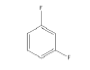3 -1.02936 -430.848 -44.5726 0.99671 12.0267 0.22974 -1.55439 -530.106 -49.319 0.99710 15.7503 0.21985 -1.55658 -530.101 -50.9781 0.99647 15.9343 0.23966 -1.54256 -530.11 -45.3645 0.99984 15.0038 0.2427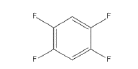7 -2.07702 -629.365 -53.4034 0.99905 15.1807 0.21598 -2.07248 -629.364 -52.176 0.99897 14.8977 0.2288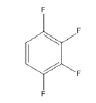9 -2.07806 -629.360 -55.0318 0.99620 17.8061 0.2284

Table 3. Calculated tEDA, Energy, ASE, HOMA, NICS and HOMO-LUMO gap of different isomers of di-, tri- and tetra-fluorinated benzene.

As shown in Table 3, di-fluorinated benzene had three isomers. Between them the ortho isomer has maximum electron removing from ring and according to prediction when the available electron in the ring decreases, the current will decreases and then the aromaticity of ring decrease. The prediction of ring aromaticity was confirmed by calculated quantity of energy, ASE and HOMA. However the NICS and HOMO-LUMO gap valued didn’t confirm the prediction. The energy, ASE and HOMA results obtained for tri-and tetra-fluorinated benzene also confirmed the aromaticity prediction for each isomer from the calculated tEDA. The exception of this is seen for tetra fluorinated benzene which molecule 7 had higher HOMA value but according to tEDA, energy and ASE the molecule 8 had highest. This fact might be as the result of symmetry effect in HOMA calculation which made the same C-C bound and consequently higher HOMA for 7. Unfortunately in this case, the NICS and HOMO-LUMO gap had no trend and no relation could be found between them and other aromaticity indices.

#### Conclusions

The main purpose of this research was to study effect of electronegative substituents on aromaticity of the substituted benzene molecules. For this reason, different substituents such as F, Cl, NO2 and CF3 were selected and used instead of H atoms of benzene. The calculated NICS which was a descriptive index for aromaticity of the ring showed interesting trends and it was demonstrated that, with increasing the substituent number, NICS value increased.

It is considerable that the NICS results had the same trend for all the substituents. It was in contradiction to prediction of the aromaticity which supposes that the electronegative substituents decrease the aromaticity of ring due to reducing in electron charge in ring. For explaining these results, effect of the substituents was calculated in the absence of ring current (in the substituted cyclohexane) and it was represented that the magnetic field in the rings was affected by the fields induced by presenting other atoms, which might be the result of the fact that magnetic fields are additive.

For the ASE result, dissimilar behaviors were seen and it was illustrated that the ring aromaticity decreased as the number of substituents increased. HOMO-LUMO energy gap calculation illustrated the same trend as the one for ASE calculation; but, the HOMA index calculation provided no valuable information. Finally, the isomerization effect was studied and it was shown that the energy, ASE and HOMA indices relate to calculated tEDA.

As the result, it was shown that the aromaticity indices relating to energy and stability of rings were more reliable in terms of interpreting aromaticity. Additionally, it was shown that the NICS values originated from two sources: one from the ring current and one from other atoms presence. This is the reason of NICS method deficiency in predicting aromaticity of substituted aromatic rings.

### Refrences

Select your language of interest to view the total content in your interested language

###### Citations : 191

Physical Chemistry : An Indian Journal received 191 citations as per Google Scholar report

#### Indexed In

• CASS
• Open J Gate
• China National Knowledge Infrastructure (CNKI)
• Cosmos IF
• Directory of Research Journal Indexing (DRJI)
• Secret Search Engine Labs